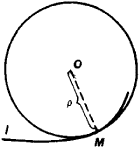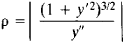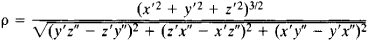# Osculating Circle

Also found in: Dictionary, Thesaurus, Wikipedia.

## osculating circle

[¦äs·kyə‚lād·iŋ ′sər·kəl]
(mathematics)
For a plane curve C at a point p, the limiting circle obtained by taking the circle that is tangent to C at p and passes through a variable point q on C, and then letting q approach p.
McGraw-Hill Dictionary of Scientific & Technical Terms, 6E, Copyright © 2003 by The McGraw-Hill Companies, Inc.
The following article is from The Great Soviet Encyclopedia (1979). It might be outdated or ideologically biased.

## Osculating Circle

(or circle of curvature). The osculating circle of a curve l at a point M is the circle having contact of order n ≥ 2 with l at M. If l has zero curvature at M, the osculating circle degenerates to a line.

Since the order of contact of the osculating circle with l is at least 2, the osculating circle reproduces the shape of l to within infinitesimals of the third order relative to the dimensions of a portion of the curve. Figure 1 shows the usual relative positions of a curve and its osculating circle; the order of contact is 2. The curve penetrates the osculating circle at M.Figure 1

The radius of curvature of l at M is the radius of the osculating circle, and the center of curvature is the center of the osculating circle. If l is a plane curve defined by the equation y = f(x), the radius of the osculating circle is given by the formulaIf l is a twisted curve defined by the equations x = x(u), y = y(u), and z = z(u), the radius of the osculating circle is given by the formulaHere, the primes indicate differentiation with respect to the parameter u. (See alsoDIFFERENTIAL GEOMETRY.)

### REFERENCE

Rashevskii, P. K. Kurs differentsial’not geometrii, 4th ed. Moscow, 1956.